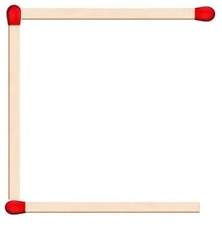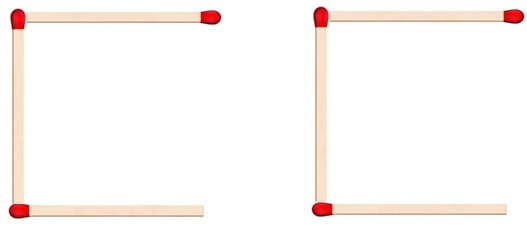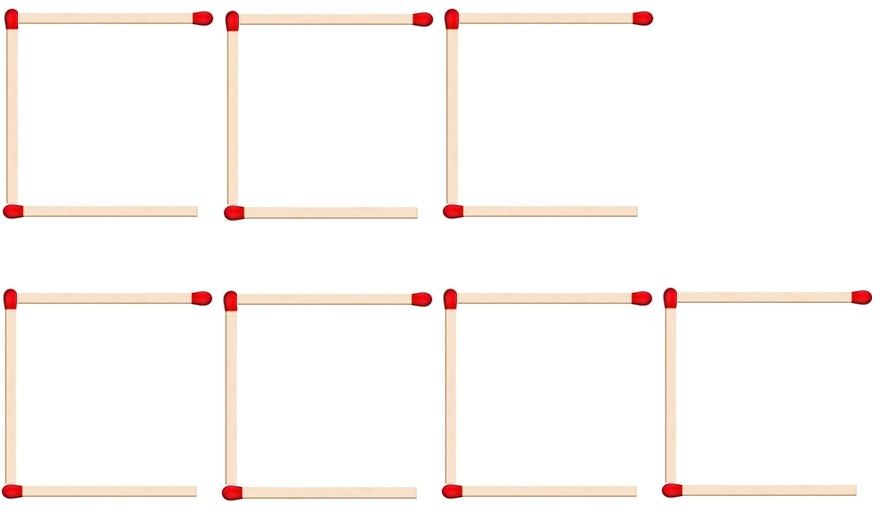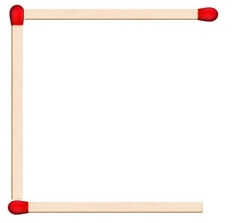Matchstick pattern

Chapter 11 Class 6 Algebra
Concept wise

Suppose we use matchsticks to form letter CHere, we used 3 matchsticks

Suppose, we want to Make more than one CSo,

2 Cs require 6 matchsticks

3 Cs require 9 matchsticks

4 Cs require 12 matchsticks

And so on………

We can say that,

Number of matchsticks = 3 × Number of C

Writing n = number of Cs

Number of matchsticks = 3nLet’s Check

For n = 1, Number of matchsticks = 3 × 1 = 3

For n = 2, Number of matchsticks = 3 × 2 = 6

For n = 3, Number of matchsticks = 3 × 3 = 9

So, Writing

Number of matchsticks = 3n

makes our job easier.

Suppose we have to make 100 Cs

Number of matchsticks = 3 × 100

= 300

Learn in your speed, with individual attention - Teachoo Maths 1-on-1 Class